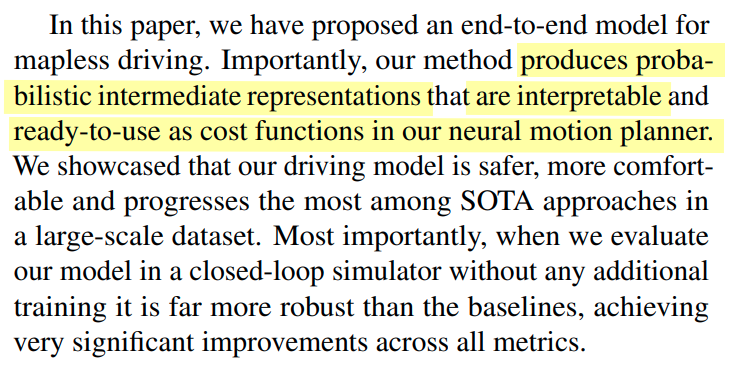# 【论文阅读】CVPR2021: MP3: A Unified Model to Map, Perceive, Predict and Plan

Sensor/组织: Uber
Summary: 非常棒！端到端输出map中间态 一种建图 感知 预测 规划的通用框架
Type: CVPR
Year: 2021

# 1. Motivation

HD Map有相当丰富的语义信息比如lanes，crosswalks，traffic lights等等的拓扑和信息。这些信息给 感知和motion forecast任务 提供了很多先验信息。但是

1. 人为制作hd map是非常cost的，而且还需要实时去根据道路维护hd map
2. 即使是持续维护hd map，在运行过程中也要保证有厘米级的定位

## 问题场景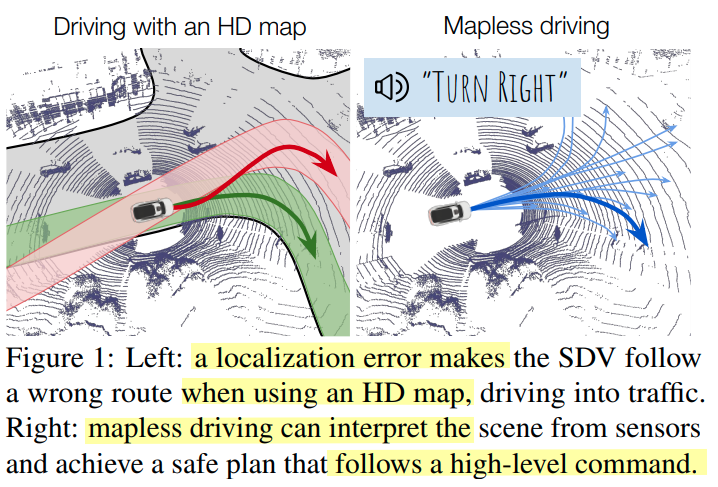1. interpretability 对于自动驾驶系统的可解释性
2. 直接的端到端形式 缺乏整体结构和先验知识的有效利用 brittle to distribution shift 

## Contribution

• 这点怎么证明？… 怎么说明没有information loss 或是其他方法有 信息损失呢？

# 2. Method

1. 提出了使用probabilistic spatial layers去建模环境内的静态和动态部分，其中
• 静态部分是以规划为中心的online mapping，提取处那些区域可以进行驾驶和相关的交通信息（比如红绿灯 限速等）
• 动态部分主要是其他道路交通参与者，用一个novel occupancy flow来提供随时间 occupancy和 其速度信息。
2. 随后走到motion planning模块进行retrieve dynamically feasible trajectories，预测地图上的spatial mask以给出SDV一条能走的路，同时使用online mapping和occupancy flow用来作为计算可解释性的safe planning cost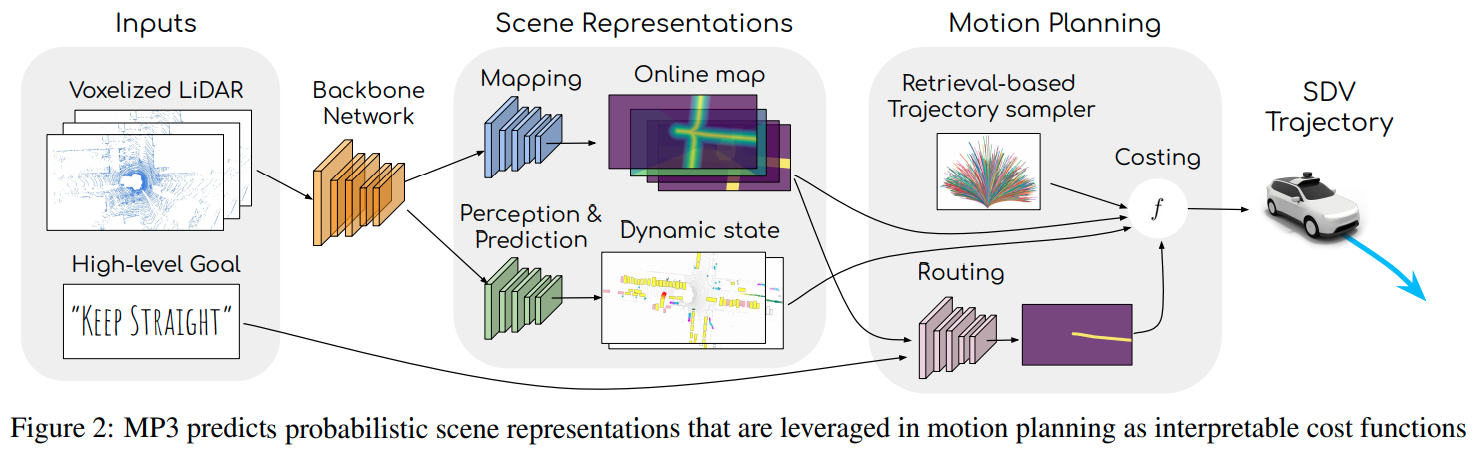## 2.1 LiDAR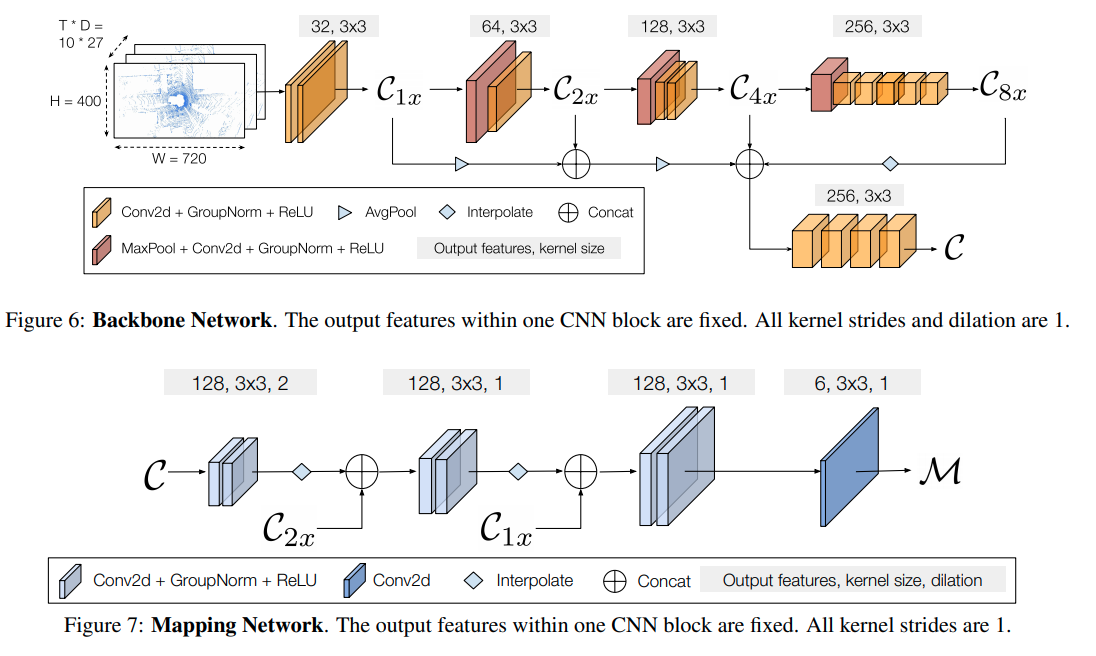## 2.2 Scene Representations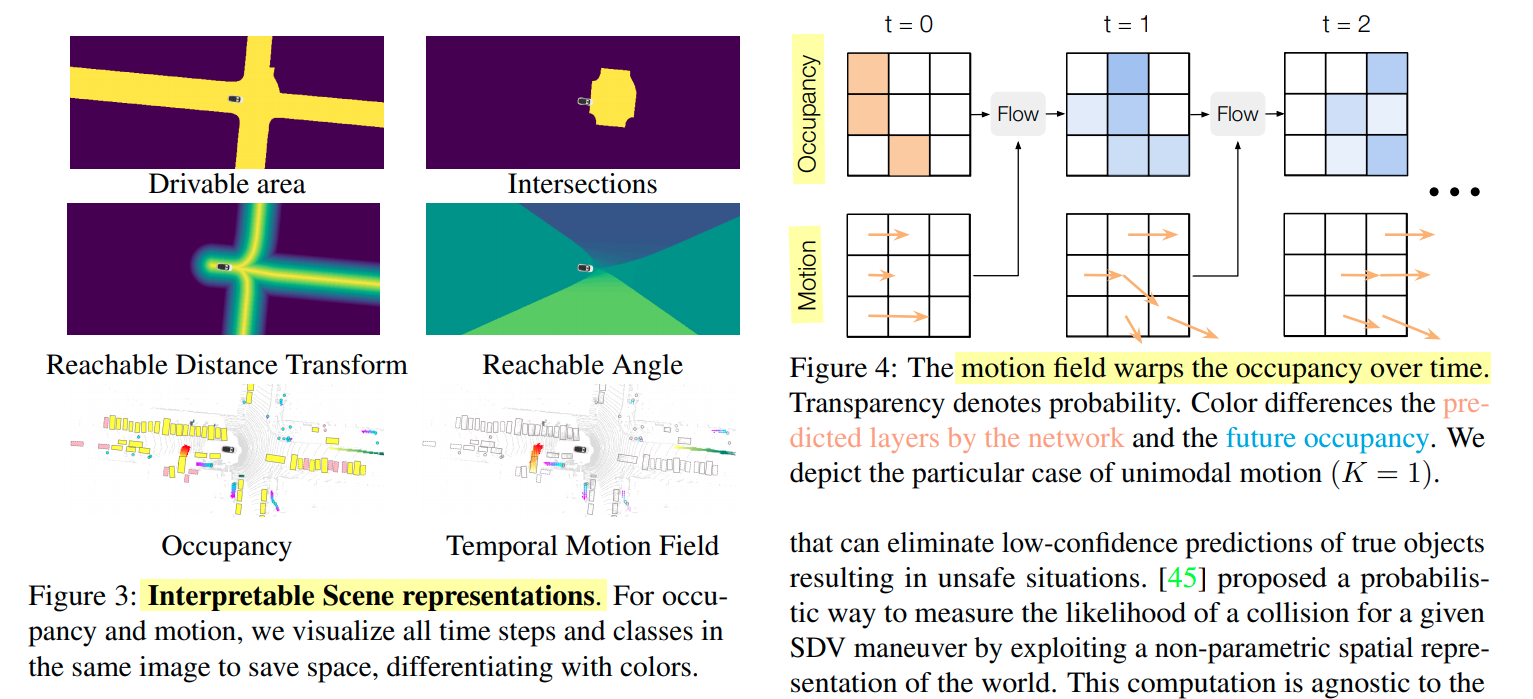online map：主要包含可行驶区域（drivable area），可到达道路（reachable lanes），交叉路口（intersection）；正常情况下 我们希望SDV尽可能接近reachable lanes，也就是道路中心线，交叉路口主要帮助理解红绿灯，停车和让行等标志

dynamic occupancy field：主要是帮助SDV理解其他道路参与者，如图四所示，先进行实际的栅格占据，再根据预测的路径进行flow的添加，BEV 0.4m/pixel 分辨率，包含：初始栅格（Initial occupancy），temporal motion field‘；需要注意的是 对 vehicles, pedestrains and bikes 进行了分类 各自都有自己的occupancy flow

### 概率模型

• 如图三所示 每个map都是自己的语义通道，定义为$$\mathcal M$$
• $$i$$ 表明 spatial index
• drivable area 和 交叉路口 通道分别用 $$\mathcal{M}_{i}^{A} \text { and } \mathcal{M}_{i}^{I}$$ Bernoulli random variables 伯努利分布
• 将SDV到车道中心线的距离model as 拉普拉斯算子 $$\mathcal{M}_{i}^{D}$$，因为作者发现比Gaussian更准确
• 将SDV到最近车道中心线的方向 表示为 $$\mathcal{M}_{i}^{\theta}$$ 用Von Mises 分布 因为范围可以从 -pi到pi
• 前面提到了 会对动态障碍物进行分类 $$\mathcal O^c$$ 其中c为class 包含：车辆、行人、骑自行车的人 $$\mathcal O^c_{t,i}$$ 为类c下 spatio temporal index t,i 的Bernoulli random variables
• 对每个类别在每个 spatio-temporal location进行建分布：$$\mathcal{K}_{t, i}^{c}$$ over K BEV motion vectors $$\left\{\mathcal{V}_{t, i, k}^{c}: k \in 1 \ldots K\right\}$$

$p\left(\mathcal{F}_{\left(t, i_{1}\right) \rightarrow\left(t+1, i_{2}\right)}^{c}\right)=\sum_{k} p\left(\mathcal{O}_{t, i_{1}}^{c}\right) p\left(\mathcal{K}_{t, i_{1}}^{c}=k\right) p\left(\mathcal{V}_{t, i_{1}, k}^{c}=i_{2}\right)$

$p\left(\mathcal{O}_{t+1, i}^{c}\right)=1-\prod_j\left(1-p\left(\mathcal{F}_{(t, j) \rightarrow(t+1, i)}^{c}\right)\right)$

## 2.3 Motion Planning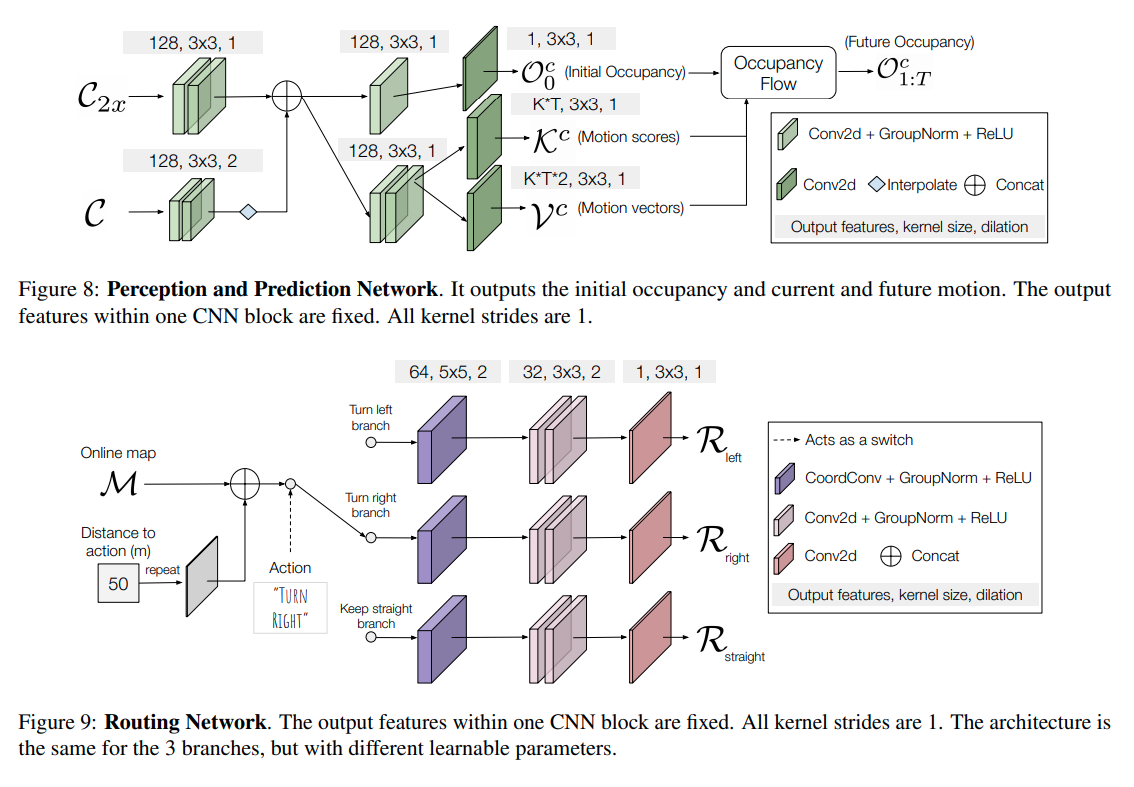Trajectory Sampling

1. 从数据集里提取出车辆轨迹，一共150个小时的manual driving data

2. 聚类（因为高效）每个轨迹的bin包含当前SDV的速度、加速度和曲率

使用初始 速度、曲率和加速度 对应每个bin size为 2.0 (m/s), 0.02 (1/m), 1.0 (m/s^2)，去将轨迹分类成不同的bins；每个bin中的轨迹都会被聚类成3000个sets，然后 closest trajectories to cluster prototype 会被保留

3. 再加上速度和曲率放入自行车模型 给一遍 rollout 来生成连续速度和转角的轨迹。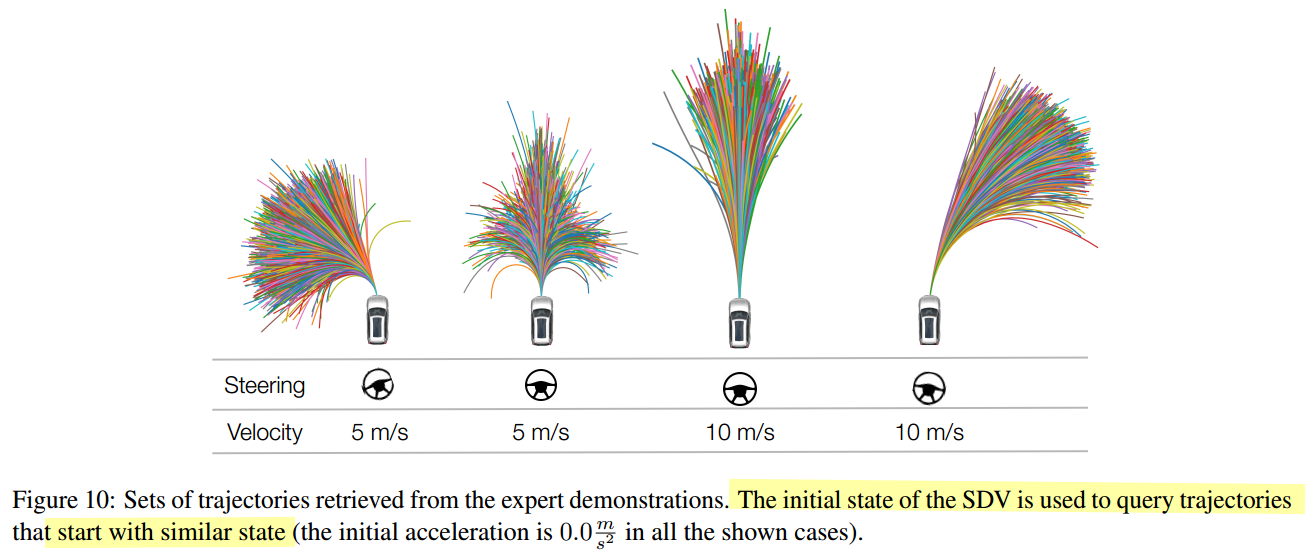**Route Prediction**

### Scoring Cost

Routing and Driving on Roads

1. 为鼓励SDV执行high-level command，使用评分函数使得SDV 在 $$\mathcal R$$ 中概率较高的区域行驶更远距离的轨迹

$f_{r}(\tau, \mathcal{R})=-m(\tau) \min _{i \in m(\tau)} \mathcal{R}_{i}$

其中 $$m(\tau)$$ 是指 BEV下 SDV选择轨迹 $$\tau$$ 所占领的grid cells。用上述 scoring function 可以确保 SDV 保持在路线上，并且仅在路线内移动时才会获得奖励。

2. 引入了一个cost-to-go，来应对超出计划范围的预测路线，对于尽头转弯或者车辆高速下比较有用。具体来说，假设 SDV 保持恒定的速度和航向，我们计算所有与 SDV 重叠的 BEV 网格单元 j 的平均值 $$1-R_j$$

3. 为确保SDV行驶在道路中心线上，use the predicted reachable lanes distance transform $$\mathcal M^D$$ 去对轨迹点进行penalize。

4. 同时为了考虑 $$\mathcal M^D$$$$\mathcal M^\theta$$ 上的不确定性，使用 cost function： product of SDV velocity and standard deviation of gird cells，即 $$\mathcal M^D$$$$\mathcal M^\theta$$ 下 SDV 所占领的那些格

$f_{d}\left(\mathbf{x}, \mathcal{M}^{\theta}, \mathcal{M}^{D}\right)=\sum_{i \in m(\mathbf{x})} \mathbf{x}_{v}\left(\sigma_{i}^{D}+\frac{1}{k_{i}^{\theta}}\right)$

其中 $$k_i^\theta$$ is the concentration parameter of the von Mises distribution representing lane direction

5. 使用一个penalize 约束SDV在道路上，不去碰撞边缘

$f_{a}(\mathbf{x}, \mathcal{M})=\max _{i \in m(\mathbf{x})}\left[1-P\left(\mathcal{M}_{i}^{A}\right)\right]$

6. 再加一个交叉路口的红绿灯cost，之间使用predicted junction probability map $$\mathcal M^J$$ 对闯红灯的行为进行惩罚

Safety

$f_{o}\left(\mathbf{x}_{t}, \mathcal{O}\right)=\sum_{c} \max _{i \in m\left(\mathbf{x}_{t}\right)} P\left(\mathcal{O}_{t, i}^{c}\right)$

Comfort

• 附录有部分公式

• $f_{d}(\mathbf{x}, \mathcal{M})=\underset{i \in m(\mathbf{x})}{\mathbb{E}}\left|\mathcal{M}_{i}^{\theta}-\mathbf{x}_{\theta}\right|$

## 2.4 Training Loss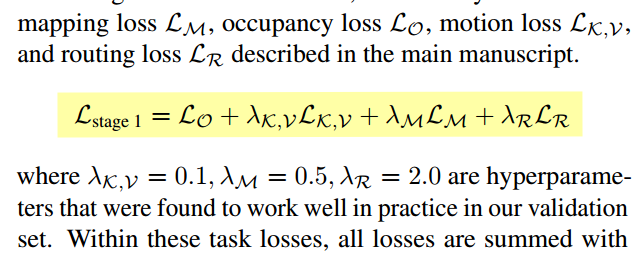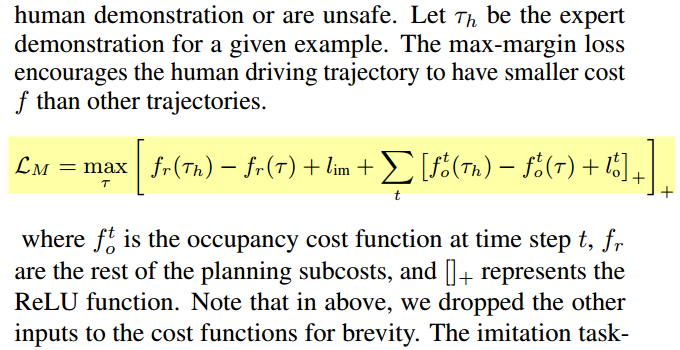# 3. 实验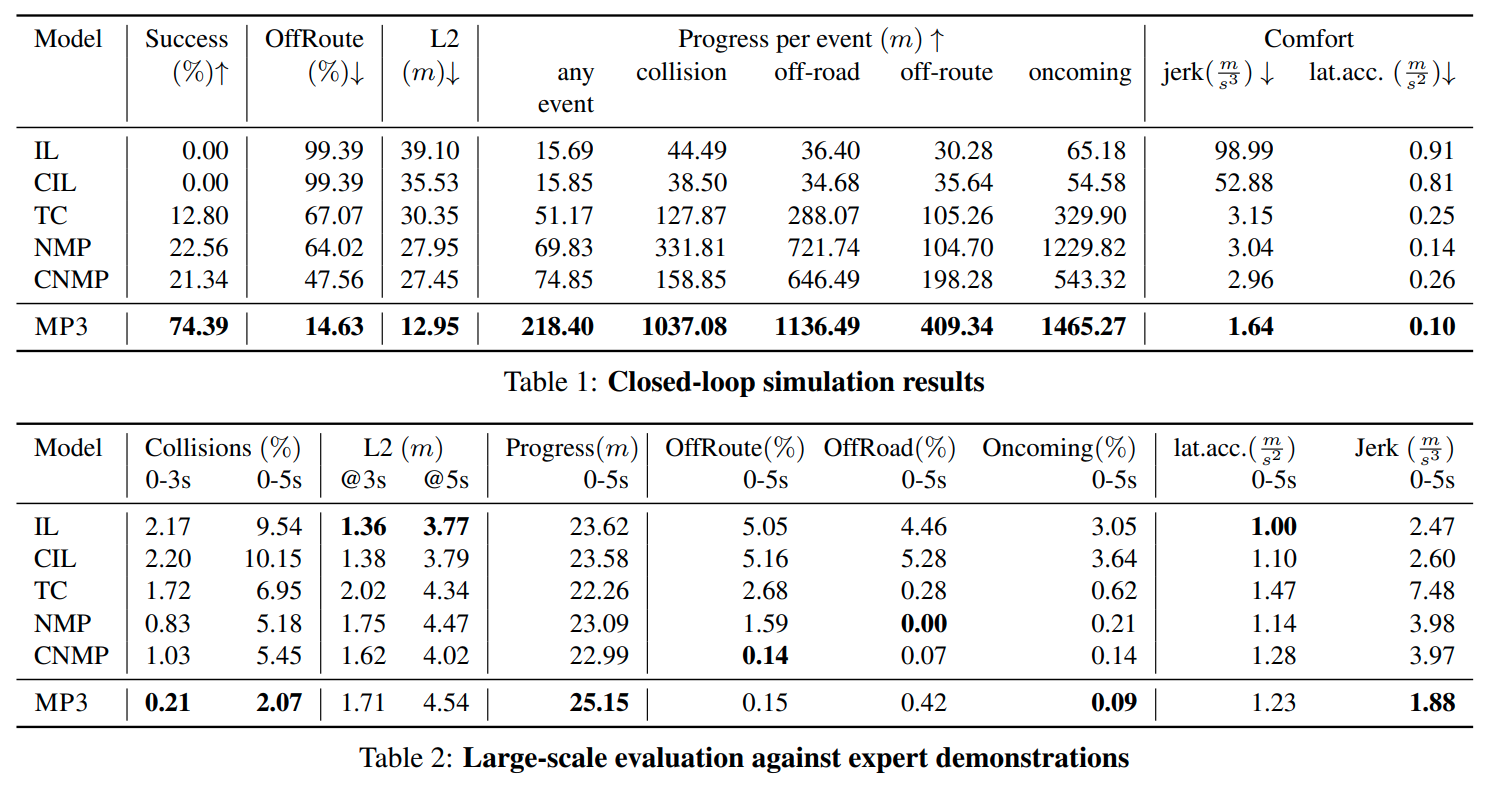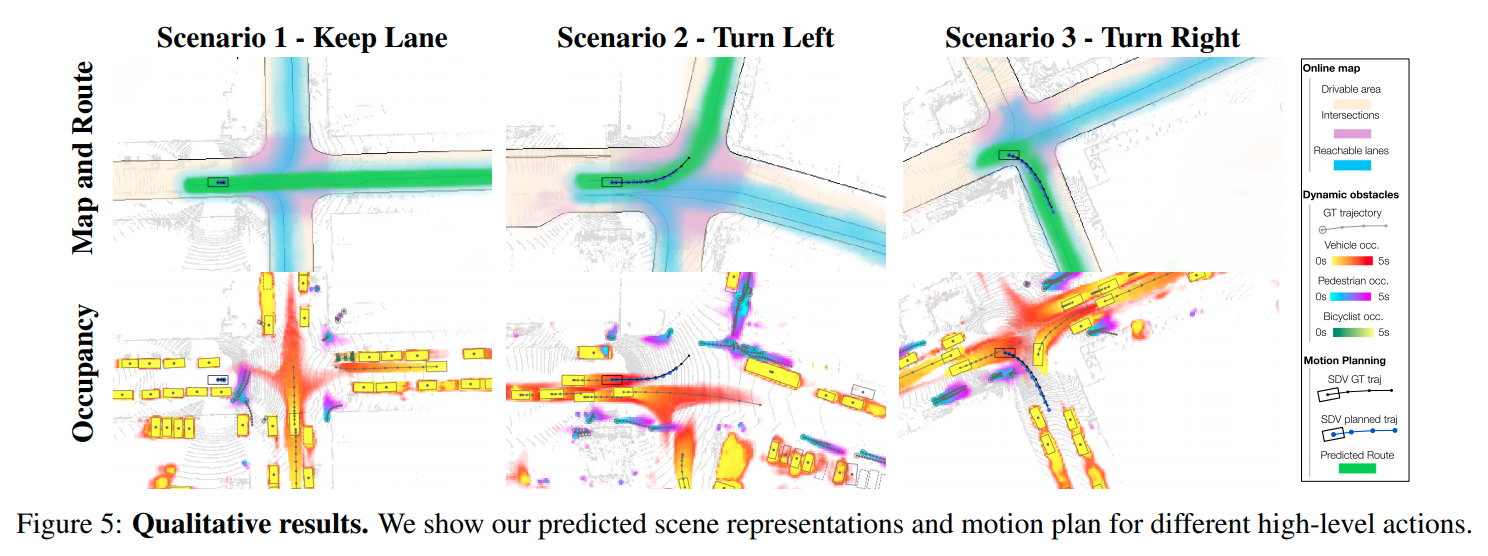# 4. Conclusion